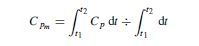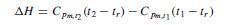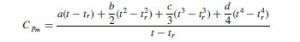# Mean Heat Capacity & Pressure Effect

The use of mean heat capacities often facilitates the calculation of sensible-heat changes; mean heat capacity over the temperature range t1 to t2 is defined by the following equation:Mean specific heat values are tabulated in various handbooks. If the values are for unit mass, calculated from some standard reference temperature, tr , then the change in enthalpy between temperatures t1 and t2 is given by:where tr is the reference temperature from which the values of Cpm were calculated.
If Cp is expressed as a polynomial of the form: Cp = a + bt + ct2 + dt3, then the integrated form of equation will be:where t is the temperature at which Cpm is required. If the reference temperature is taken at 0 degree C:

and the enthalpy change from t1 to t2 becomes

THE EFFECT OF PRESSURE ON HEAT CAPACITY

The data on heat capacities given in the handbooks, and in Appendix A, are, usually for the ideal gas state. Equation 3.13a should be written as:

C’p = a + bt + ct2 + dt3

where the superscript ( ‘ ) refers to the ideal gas state.
The ideal gas values can be used for the real gases at low pressures. At high pressures the effect of pressure on the specific heat may be appreciable.
Edmister (1948) published a generalised plot showing the isothermal pressure correction for real gases as a function of the reduced pressure and temperature. His chart, converted to SI units, is shown as Figure 3.2. Edmister’s chart was based on hydrocarbons, but can be used for other materials to give an indication of the likely error if the ideal gas specific
heat values are used without corrections.